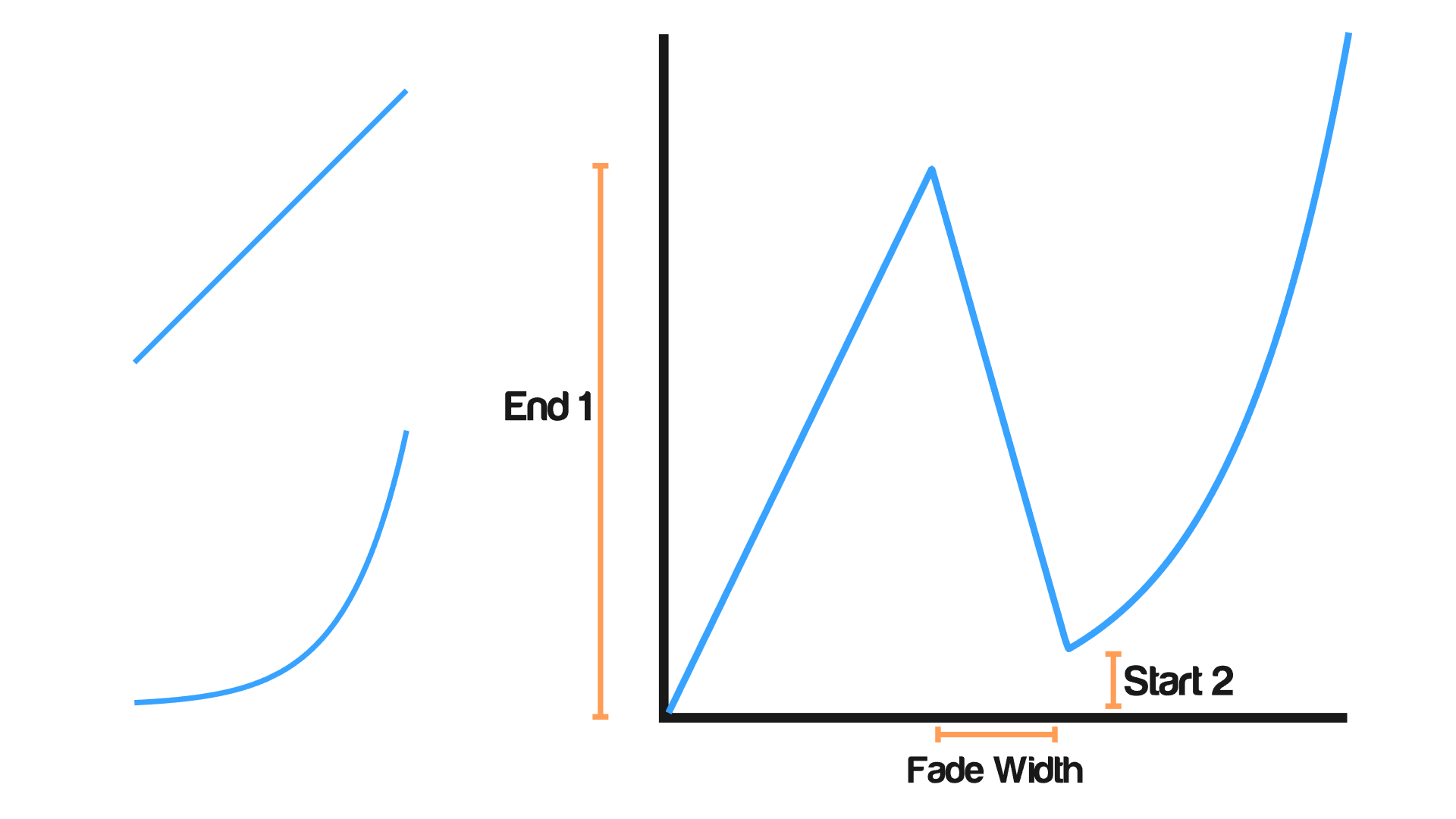# Mix Interpolation

## Description

This node mixes between two interpolations by a defined factor.

## Options

• Overlay - In this option, if one were to mix two interpolations by a factor `F` and evaluate the output interpolation at the value `V`, He would observe that the result is identical to the result of evaluating both interpolations at `V` and mixing their outputs by the factor `F`.
• Chain - This option stack input interpolations into a single interpolation. The first interpolation will be used when evaluating at values from zero to input Position while the second will be used when evaluating at values from there to 1, that is of course after they get compressed from 0,1 range to those smaller intervals. Here is an illustration for mixing a linear interpolation with an exponential one:## Inputs

• Interpolation 1 - An interpolation.
• Interpolation 2 - An interpolation.
• Factor - A float that control the amount of each interpolation input to the output, Where 0 means the first interpolation only and 1 means the second interpolation only. (Only in overlay option)
• Position - The position at which the first interpolation ends and the second interpolations starts assuming that the fade width is set to zero. (Only in chain option)
• End 1 - It is the maximum value of the first interpolation. (Only in chain option)
• Start 2 - It is the minimum value of the second interpolation. (Only in chain option)
• Fade Width - It is the difference between the end of the first interpolation and the start of the second interpolation. (Only in chain option)

## Outputs

• Interpolation - The result of mixing the two interpolations.